# The Total Expenses Formula: How Much Is A Company Spending?

If you want to know how much a company is spending, you can use the total expenses formula. This blog post will show you how to use this important equation.If you’ve ever run a business or even just managed your own finances, you know that one of the most important things to track is your business expenses. After all, it’s not enough to simply know how much money is coming in – you also need to know where it’s going. Otherwise, you might find yourself in a situation where you’re spending more than you’re making and end up in debt. That’s why it’s so important to have a good handle on the total expenses formula. This simple accounting equation will help you calculate a business’s operating expenses and give insights into its financial health. So whether you’re trying to keep tabs on your own spending or want to see how well a company is doing, the total expenses formula is an essential tool.

## Total Expenses Formula

The total expenses formula is used to calculate the total amount of money that a company has spent over a period of time. This information is important for financial planning and analysis. The formula is used to track expenses patterns and identify areas where cost savings can be made.

## Total Expenses – How Much Is A Company Spending?

A company’s expenses can give us valuable insights into its spending habits over time. By tracking a company’s expenses, we can see how it is allocating its resources and where it may be cutting corners. This information can be useful for both investors and managers alike.

A company’s revenues are its income.

As an investor, you will notice that there are several expenses being subtracted from a company’s total revenue. These expenses include the cost of goods sold, research and development, selling, general and administrative, depreciation, and other miscellaneous expenses. All of these expenses must be accounted for in order to calculate a company’s net income.

After we subtract the aforementioned expenses, we are left with EBIT. To calculate Net Income, we will then subtract Interest and Taxes from EBIT.

It just means that the company is investing more in its operations.

It is highly likely that expenses will increase along with a company’s revenue. After all, it does take money to make money.

What are some possible explanations for a manufacturer’s expenses increasing, even when revenue streams aren’t?

This metric is used to compare a company’s spending over time. Comparing expenses to competitors can be tricky, but it can still be useful.

The total amount of expenses for a quarter is the total revenues for that same quarter minus the net earnings for that same period.

## What is the Operating Expense Formula?

Operating expenses are the costs associated with running a business that are not directly related to production costs goods or services. These costs can include rent, marketing, employee wages, and other general overhead expenses.

So, it’s known as operational expenses or “OPEX.”

Operating Expense = Total Revenue – Total Cost of Goods Sold

Operating expenses are calculated by subtracting a company’s operating income from its total revenue. Mathematically, this is represented as total revenue minus operating income (EBIT).

The operating expense formula is used to calculate a company’s total operating expenses for a given period of time. Operating expenses are all the costs associated with running a business, excluding the cost of goods sold. The formula is: Operating Expenses = Cost of Goods Sold + Selling, General, and Administrative Expenses To calculate a company’s operating expenses, you will need to know the cost of goods sold and the selling, general, and administrative expenses. These can be found on a company’s income statement.

### Examples of Operating Expense Formula (With Excel Template)

Let’s use an example of calculating operating expenses to better understand it.

#### Operating Expense Formula – Example #1

The company’s operating expenses can be calculated using the following formula: Operating Expenses = Total Revenue – Cost of Goods Sold – Gross Profit Therefore, based on the information provided, the company’s operating expenses would be: Operating Expenses = \$1,000,000 – \$500,000 – \$200,000 Operating Expenses = \$300,000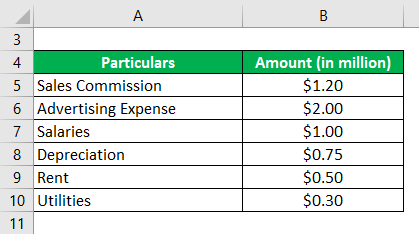(Source)

Solution:

Calculate the company’s operating expenses based on the information provided.

The operating expense is calculated as:

The cost of doing business = the cost of sales commission + the cost of advertising expense + the cost of salaries + the cost of depreciation + the cost of rent + the cost of utilities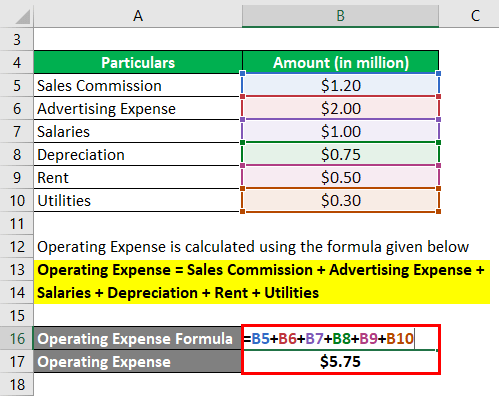(Source)

Operating Expense is the total of all expenses incurred while running a business. In this example, the Operating Expense is \$5.75 million. This includes expenses such as labor, rent, materials, and other costs associated with running the business.

The operating expenses for the company during the 6 months were \$5.75 million dollars.

#### Operating Expense Formula – Example #2

Let’s use an example to illustrate an alternate approach to computing operating expense. Let’s say a company has the following financial information: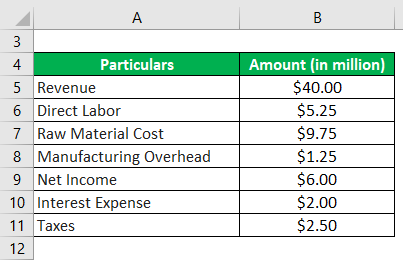(Source)

The solution:

The formula for calculating the Cost of Goods Sold is:

COGs = Cost of Goods Sold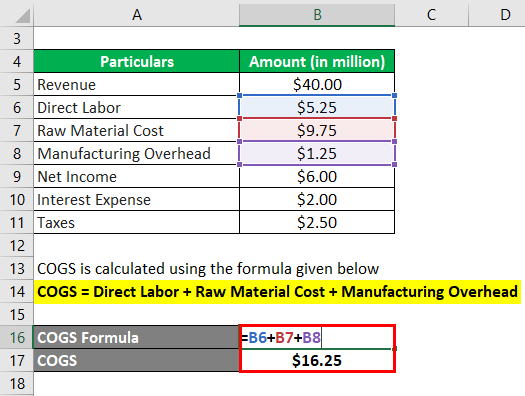(Source)

The cost of goods sold (COGS) for the company is \$16.25 million. This includes the costs of materials, labor costs, and overhead associated with the production process of goods.

The formula for calculating your operating profitability is:

The formula for calculating a company’s operating income (EBIT) is net income plus interest expense plus taxes.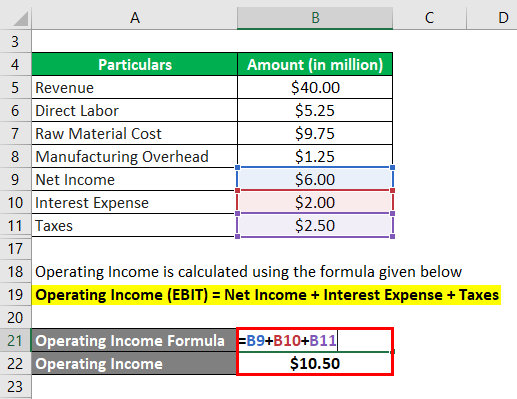(Source)

Operating Income = \$6.00 million + \$2.00 million + \$2.50 million This gives us an operating income of \$10.50 million.

The operating expense is calculated as:

Operating Expense = Revenue – (Revenue – COGS) Operating Expense = COGS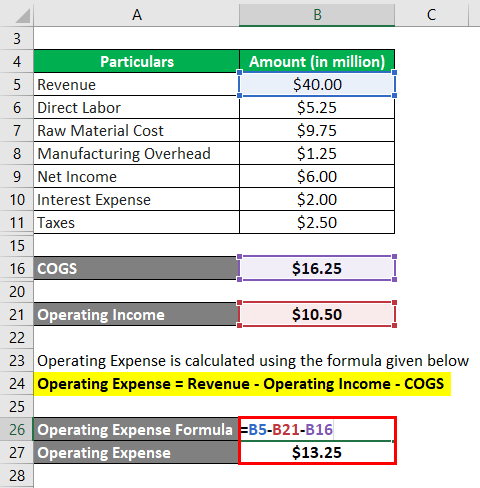(Source)

Operating Expense is calculated to be \$13.25 million by taking the total of \$40.00 million and subtracting both the cost of goods sold at \$10.50 million as well as the overhead expenses at \$16.25 million.

The operating expenses of this company during the 2016 fiscal year were \$13.25 million dollars.

#### Operating Expense Formula – Example #3

Apple Inc. is a good example to illustrate how the calculation of operating expenses changes when you use different approaches.

Revenue: \$100,000 Expenses: \$75,000 Net Income: \$25,000 The company’s revenue is \$100,000, their expenses are \$75,000, and their net income is \$25,000.(Source)

The solution:

First Approach

The operating expense is calculated using the following formula:

The operating expense is the sum of research and development expenses, and selling, general and administrative expenses.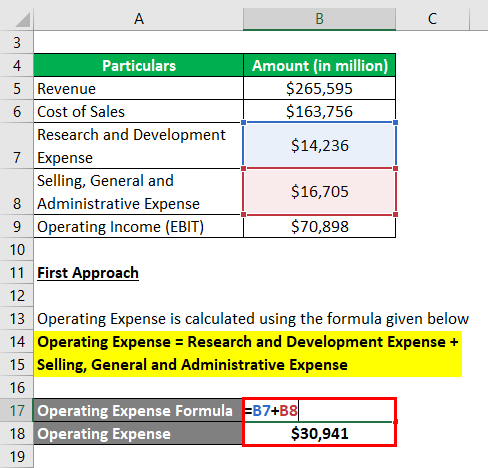(Source)

Operating Expense for the company is \$30,941 million. This is calculated by adding \$14,236 million and \$16,705 million.

### Relevance and Use of Operating Expense Formula

Operating expenses are an important part of assessing a company’s financial health because they can indicate how profitable the company is.

Since these costs are recurring and not related to production, most companies try to minimize them in order to boost their profit margins.

Operating expenses play an important role in decision making as they can help build competitive advantage. The outcome of these decisions can be used to assess the performance of management, which reflects their ability to take initiatives and make competent decisions.

## Conclusion

The total expenses formula is a simple but essential tool for anyone trying to keep track of their spending or assess the financial health of a company. By understanding how to calculate operating expenses, you can get valuable insights into where your money is going and make more informed decisions about your finances.